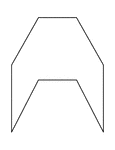### Irregular Concave Octagon

Illustration of an irregular concave octagon. This polygon has some symmetry.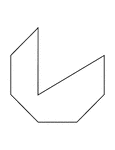### Irregular Concave Octagon

Illustration of an irregular concave octagon.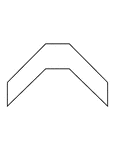### Irregular Concave Octagon

Illustration of an irregular concave octagon. This polygon has some symmetry.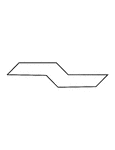### Irregular Concave Octagon

Illustration of an irregular concave octagon. This polygon has some symmetry.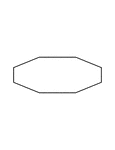### Irregular Convex Octagon

Illustration of an irregular convex octagon. This polygon has some symmetry.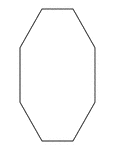### Irregular Convex Octagon

Illustration of an irregular convex octagon. This polygon has some symmetry.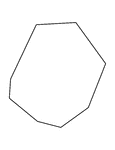### Irregular Convex Octagon

Illustration of an irregular convex octagon.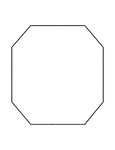### Irregular Convex Octagon

Illustration of an irregular convex octagon. This polygon has some symmetry.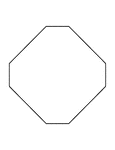### Irregular Convex Octagon

Illustration of an irregular convex octagon. This polygon has some symmetry.